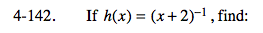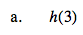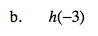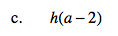### Home > AC > Chapter 13 > Lesson 13.CS1-S > Problem4-142

4-142.h(3) = (3 + 2)−1 = 5−1

$h(3)=\frac{1}{5}$h(−3) = (−3 + 2)−1 = (−1)−1See part (b).